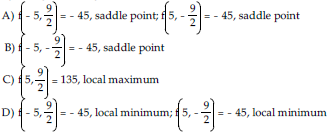/
/
/
221) Find the equation for the tangent plane to the
Not my Question
Flag Content

# Question : 221) Find the equation for the tangent plane to the : 1757203

221) Find the equation for the tangent plane to the surface z = ln(7x2 + 3y2 + 1) at the point (0, 0, 0).

A) x + y + z = 0 B) x - y = 0 C) z = 0 D) x + y = 0

222) Find the equation for the tangent plane to the surface z = e4x2 + 8y2 at the point (0, 0, 1).

A) z = 0 B) z = 2 C) z = 1 D) z = -1

223) f(x, y) = -2x - 7y + 6 at (9, 6)

A) L(x, y) = -18x - 42y + 6 B) L(x, y) = 9x + 6y + 6

C) L(x, y) = -18x - 42y + 36 D) L(x, y) = -2x - 7y + 6

224) f(x, y) = 10x2 + 4y2 - 10 at (9, 2)

A) L(x, y) = 20x + 8y - 836 B) L(x, y) = 180x + 16y + 816

C) L(x, y) = 20x + 8y + 816 D) L(x, y) = 180x + 16y - 836

225) f(x, y) = 9x2y3 at (-3, -9)

A) L(x, y) = -13,122x + 19,683y + 236,196 B) L(x, y) = 39,366x + 19,683y + 236,196

C) L(x, y) = 39,366x - 6561y + 236,196 D) L(x, y) = -13,122x - 6561y + 236,196

226) f(x, y) = e4x + 8y at (0, 0)

A) L(x, y) = 8x + 4y B) L(x, y) = 4x + 8y + 1

C) L(x, y) = 4x + 8y D) L(x, y) = 8x + 4y + 1

227) f(x, y) = 7 sin x + 5 cos y at (0, π/2)

A) L(x, y) = 7x - 5y + 5/2 π B) L(x, y) = 7x + 5y + 5/2 π

C) L(x, y) = -7x + 5y + 5/2 π D) L(x, y) = -7x - 5y + 5/2 π

228) f(x, y) = x2 + 10x + y2 - 4y - 7

A) f(-5, 2) = -36, local minimum B) f(-5, -2) = -20, saddle point

C) f(5, 2) = 64, saddle point D) f(5, -2) = 80, local maximum

229) f(x, y) = 2xy + 9x + 10y230) f(x, y) = -10xy(x + y) + 6

A) f(0, 0) = 6, saddle point; f(-10, -10) = 20,006, local maximum

B) f(-10, -10) = 20,006, local maximum

C) f(-10, 0) = 6, local minimum; f(0, -10) = 6, local minimum

D) f(0, 0) = 6, test is inconclusive

## Solution 5 (1 Ratings )

Solved
Mathematics 3 Years Ago 56 Views